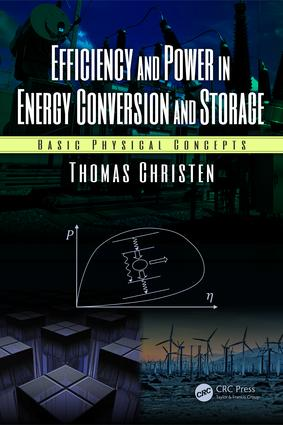Efficiency and Power in Energy Conversion and Storage

Basic Physical Concepts, 1st Edition

CRC Press

168 pages | 6 Color Illus. | 44 B/W Illus.

Hardback: 9781138626638
pub: 2018-10-23
\$159.95
x
eBook (VitalSource) : 9780429454288
pub: 2018-10-10
from \$28.98

FREE Standard Shipping!

Description

This book provides fundamental theoretical concepts for the understanding, the modelling, and the optimisation of energy conversion and storage devices. The discussion is based on the general footing of efficiency-power relations and energy-power relations (Ragone plots). Efficiency and Power in Energy Conversion and Storage: Basic Physical Concepts, is written for engineers and scientists with a bachelor-degree level of knowledge in physics. It contains:

• An introductory motivation of the topic
• A review on equilibrium thermodynamics
• A primer to linear non-equilibrium thermodynamics and irreversible processes
• An introduction to endo-reversible thermodynamics
• The basics on the theory of Ragone plots
• Derivations of efficiency-power relations or Ragone plots for illustrative examples like heat engines, batteries, capacitors, kinetic energy storage devices, solar power, photodiodes, electro-motors, transformers, and flow turbines
• An excursion to impedance matching and the optimization of technical devices with respect to economic and related objectives

Reviews

"The book is a fundamental contribution towards an efficient use of energy resources."

--Dr. Alfred Rufer, Ecole Polytechnique Federale de Lausanne

Introduction. Ragone Plots. Thermodynamics Basics. Energy, Entropy, and Efficiency. Entropy Production Rate. Endoreversible Thermodynamics. Entropy Generation Minimization. Efficiency-Power Relations. The Endoreversible Carnot Engine. Thermal Heat Storage. Battery Capacitor. Kinetic Energy Storage Devices. Electro-Motor. Super-Capacitor With Frequency Dependent Impedance. Piezoelectric Energy Harvester. Economic Optimization. Net Present Value. Applications. Other Power Maximization Problems. Wind Turbine. Photovoltaics. Solar Power.

This textbook provides fundamental theoretical concepts for the understanding, the modelling, and the optimisation of energy conversion and storage devices. The discussion is based on the general footing of efficiency-power relations and energy-power relations (Ragone plots). The book is written for engineers and scientists with a bachelor-degree level of knowledge in physics. It contains:

• an introductory motivation of the topic
• a review on equilibrium thermodynamics
• a primer to linear non-equilibrium thermodynamics and irreversible processes
• an introduction to endo-reversible thermodynamics
• the basics on the theory of Ragone plots
• derivations of efficiency-power relations or Ragone plots for illustrative examples like heat engines, batteries, capacitors, kinetic energy storage devices, solar power, photodiodes, electro-motors, transformers, and flow turbines
• an excursion to impedance matching and the optimization of technical devices with respect to economic and related objectives

All concepts and models are reduced to their basics in order to allow non-experts to easily grasp the essentials.

Thomas Christen (PhD ‘94 in theoretical physics) works as a senior principal scientist in Corporate Research of ABB Ltd, Switzerland. He periodically lectures on physics in industry at the University of Basel.